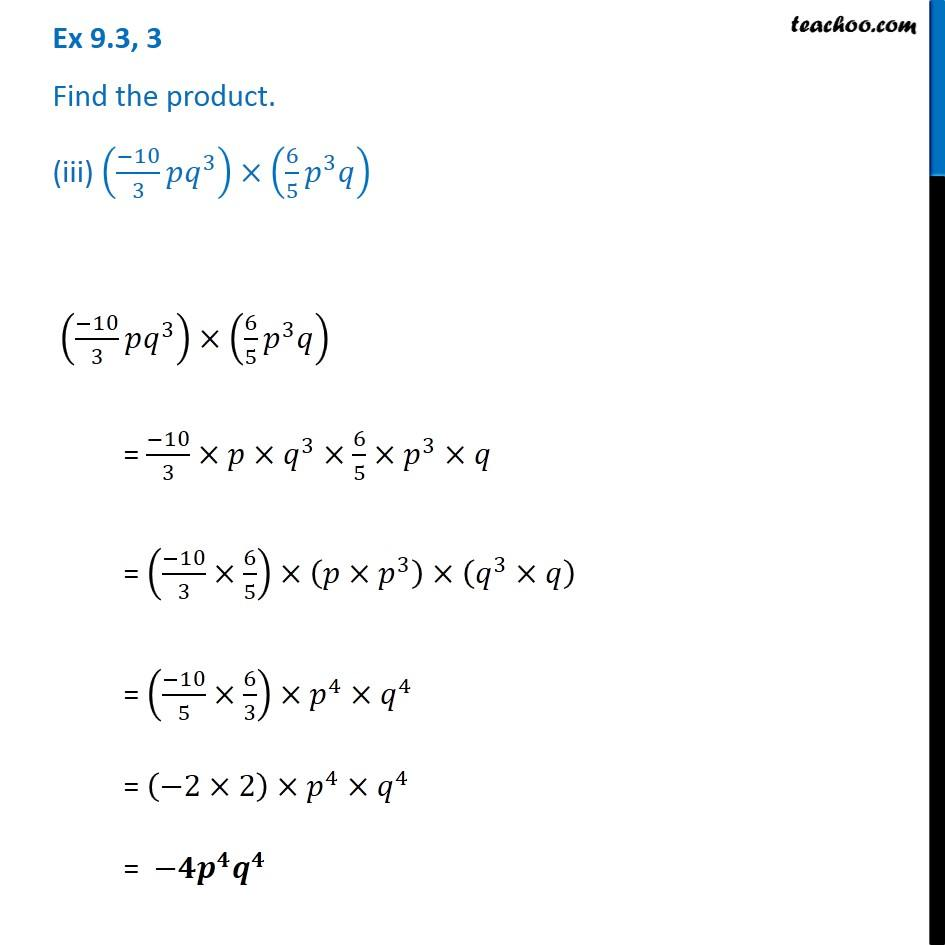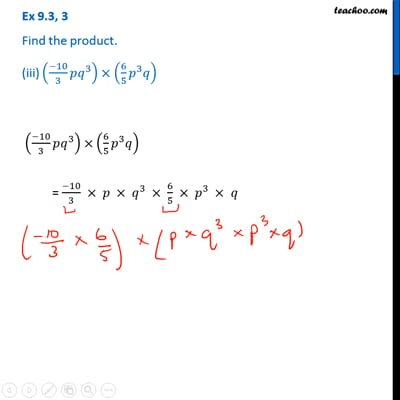Ex 9.3

Chapter 9 Class 8 Algebraic Expressions and Identities
Serial order wiseThis video is only available for Teachoo black users

Get live Maths 1-on-1 Classs - Class 6 to 12

### Transcript

Ex 9.3, 3 Find the product. (iii) ((−10)/3 𝑝𝑞^3 )×(6/5 𝑝^3 𝑞) ((−10)/3 𝑝𝑞^3 )×(6/5 𝑝^3 𝑞) = (−10)/3×𝑝×𝑞^3×6/5×𝑝^3×𝑞 = ((−10)/3×6/5)×(𝑝×𝑝^3 )×(𝑞^3×𝑞) = ((−10)/5×6/3)×𝑝^4×𝑞^4 = (−2×2)×𝑝^4×𝑞^4 = −𝟒𝒑^𝟒 𝒒^𝟒